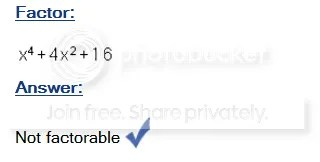Categories

# Factoring – Sending Out the Bat Signal!

One of the joys of my job is having mathematically interesting chats with my colleagues about how they approach  specific problems with their classes.  These conversations often begin as one-on-one discussions, but usually evolve into calling multiple people into the fray to give their two cents.  This semester, a teacher in my department is tackling an Accelerated Algebra II class for the first time.  Having taught academically talented kids for many years, my advice to him was to constantly challenge his students, perhaps using problems like those from the American Mathematics Competitions as openers.  But while offering up academic challenges can keep a teacher’s mind sharp, there is the risk of having that “hmmmm…” moment…..that uncomfortable feeling where you’re not quite sure what the correct response to a student question is.

The discussion today came from a review of factoring, and a problem which seems innocent enough:

#### Factor x6– 64

Take a moment and think about how you would factor this….show all work for full credit…

Enjoy a few lines of free space as you consider your work….

And…time….pencils down….

The interesting aspect of x6– 64 is that it is both a difference of cubes and a difference of squares.  I used the neat algebraic interface on purplemath.com to do some screen captures and make the algebra look pretty here.  In this case, the calculator factors this expression as a difference of squares, (x3– 8 ) (x3 + 8),  which then become both a sum and difference of cubes and can both factor further:But, the initial expression is also a difference of cubes, and can be factored as such.  It is verified below:The plot thickens as the discussion then centers about the “remnants” we get when we factor a difference of cubes.  We can verify that the two “remnants” (underlined in red) from the first factorization are factors of the remnant of the second method (underlined in green):So, what’s happening here?

The extra, messy, factor we get when we factor a sum or difference of cubes is up for discussion here.
According to Purplemath:

The quadratic part of each cube formula does not factor, so don’t attempt it.

But we don’t have a quadratic here (though we could perform a quick substitution and consider it is one), we have a 4th degree polynomial.  Even the algebra calculator on the site doesn’t care for this quirky 4th power expression:So, I am looking to my math peeps for some thoughts:

1. Is there an order to consider when a polynomial meets 2 special cases?  Should we look at sum of cubes or squares first?
2. Does anyone have any insight on x4+4x2 + 16?

Good night, and good factoring…## By Bob Lochel

HS Math Teacher. Hatboro-Horsham School District, Horsham, PA.

## 3 replies on “Factoring – Sending Out the Bat Signal!”Jon Nycesays:

I love this problem. I always use it in my Hon Alg II class. I always make sure to factor it as a difference of squares and also a difference of cubes. Students (hopefully) come to the realization, by comparing the two different factorings, that x^4+4x^2+6 = (x^2-2x+4)(x^2+2x+4). I tell them that by looking at the two different factorings it is apparent that x^4+4x^2+6 is factorable, however, we have no methods by which to factor it (other than the one we just used). I am sure that there are other polynomials that exist which are factorable, but for which we have no methods to factor. Unless someone knows of other factoring methods of which I am not aware. The rational root theorem and Descarte’s Rule of signs don’t work because the roots of x^4+4x^2+6 are all imaginary.Sue Ann Buttssays:

When applying transformations to the graphs of basic functions, one is to reflect, then dilate and then translate. Perhaps there is an order that should also be applied to an expression in which one can both find the diff of squares and also the difference of cubes. I would think one should take the squares first and then the cubes since it will generate the abilty to apply both.

It seems there just is some sort of special factoring pattern for even powered expressions here (similar to how difference of squares factors as (x-c)(x+c)) where the basic x^4 + ax^2 + (a)^2 just has a special factoring pattern as (x^2+(a/2)x + a)(x^2 – (a/2)x + a) in the same fashion that the difference of squares is just a factoring pattern. It leads me to believe that there would be some sort of factoring pattern for greater even powered expressions. Although now that I try it out, it seems as though the only reason this particular instance works out is because 2 squared is 4 but also 2 if half of four. This doesn’t work for other numbers squaring and doubling so it seems as though we have a lone case. That’s as much time as I have to think about it right now!